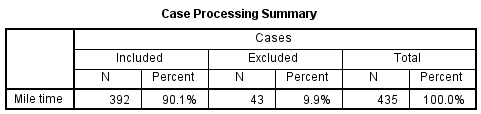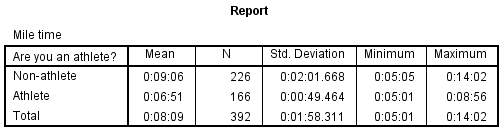# SPSS Tutorials: Descriptive Stats by Group (Compare Means)

Compare Means is best used when you want to compare several numeric variables with respect to one or more categorical variables. It is especially useful for summarizing numeric variables simultaneously across categories.

## Compare Means

The Compare Means procedure is useful when you want to summarize and compare differences in descriptive statistics across one or more factors, or categorical variables.

To open the Compare Means procedure, click Analyze > Compare Means > Means. (In SPSS version 29, the menu labeling has changed to Analyze > Compare Means and Proportions > Means.)A Dependent List: The continuous numeric variables to be analyzed. You must enter at least one variable in this box before you can run the Compare Means procedure.

B Independent List: The categorical variable(s) that will be used to subset the dependent variables. Specifying multiple values in the "Layer 1 of 1" box will produce several tables, each with one layer variable. You can specify several layers for a single table by clicking Next and then entering other categorical variables; this will produce a table that looks like a hybrid of a crosstab and the Descriptives procedure.

C Options: Opens the Means: Options window, where you can specify the summary statistics to produce, and what order they should be listed in.The Statistics column on the left shows what statistics are available. Summary statistics available include: mean, number of cases, standard deviation, median, grouped median, standard error of mean, sum, minimum, maximum, range, first, last, variance, kurtosis, standard error of kurtosis, skewness, standard error of skewness, harmonic mean, geometric mean, percent of total sum, and percent of total N. The Cell Statistics column on the right are the statistics that will be produced in the output. By default, the mean, number of cases, standard deviation will be computed. You can add additional statistics by clicking and dragging them from the Statistics column to the Cell Statistics column. You can also click and drag items in the Cell Statistics column to change the order they appear in the output.

The Statistics for First Layer area includes options that will perform one-way ANOVA and compute linear fit statistics (R, R2, Eta, and Eta Squared), respectively.

## Example: Comparing averages across related demographic variables

### Problem Statement

Running speed and ability is known to be correlated with both physical sex and with a person's general level of athleticism.

In the sample dataset, there are several variables relating to this question:

• Gender - The person's physical sex (Male or Female)
• Athlete - Are you an athlete? (Yes/No)
• MileMinDur - Time to run a mile (as a duration variable, hh:mm:ss)

Let's use the Compare Means procedure to summarize the relationship between running ability, athletics, and gender.

### Compare Means: Basic Report, No Layers

First, we will summarize the mile times without the grouping variables using the mean, standard deviation, sample size, minimum, and maximum.

#### Running the Procedure

##### Using the Compare Means Dialog Window
1. Open Compare Means (Analyze > Compare Means > Means).
2. Double-click on variable MileMinDur to move it to the Dependent List area.
3. Click Options to open the Means: Options window, where you can select what statistics you want to see. Mean, Number of Cases, and Standard Deviation are included by default. Click and drag Minimum and Maximum to the Cell Statistics box. You can also drag the items within the Cell Statistics box to change the order that the statistics are displayed in the output. Click Continue when finished.
4. Click OK.
##### Using Syntax
MEANS TABLES=MileMinDur
/CELLS=MEAN COUNT STDDEV MIN MAX.

#### Output

The Compare Means procedure will report two tables: the Case Processing Summary, which contain information about the number of valid cases that the statistics are based on, and the Report table, which contains the descriptive statistics themselves.The average mile time overall was 8 minutes, 9 seconds, with a standard deviation of about 2 minutes. The fastest mile time was about 5 minutes; the slowest was about 14 minutes.

### Compare Means: Report with One Layer

Now let's look at how the mile times vary with respect to whether or not someone is an athlete.

Note that Compare Means with one layer produces results that are similar to using the Split File technique with the Descriptives procedure. The major difference between using Compare Means and viewing the Descriptives with Split File enabled is that Compare Means does not treat missing values as an additional category -- it simply drops those cases from the analysis. Compare Means is limited to listwise exclusion: there must be valid values on each of the dependent and independent variables for a given table.

#### Running the Procedure

##### Using the Compare Means Dialog Window

If you are continuing the example from the first section, you will only need to do step 3.

1. Open Compare Means (Analyze > Compare Means > Means).
2. Double-click on variable MileMinDur to move it to the Dependent List area.
3. Click on variable Athlete and use the second arrow button to move it to the Independent List box.
4. Click Options to open the Means: Options window, where you can select what statistics you want to see. Mean, Number of Cases, and Standard Deviation are included by default. Click and drag Minimum and Maximum to the Cell Statistics box. You can also drag the items within the Cell Statistics box to change the order that the statistics are displayed in the output. Click Continue when finished.
5. Click OK.
##### Using Syntax
MEANS TABLES=MileMinDur BY Athlete
/CELLS=MEAN COUNT STDDEV MIN MAX.

#### Output

The Case Processing Summary table shows how many cases had nonmissing values for both the mile time and the athlete indicator variable. The Report table has the descriptive statistics with respect to each group, as well as the overall average mile time of the valid cases (n = 392).From this table, there are several observations we can make about the relationship between mile time and athletics in the sample:

• The sample had more non-athletes (n = 226) than athletes (n = 166).
• The fastest mile times for athletes and non-athletes were actually very close (just over 5 minutes). However, the slowest mile time was much slower for the non-athletes (14 minutes) than it was for the athletes (just under 9 minutes).
• The mean mile time for athletes was about two minutes faster than the mean mile time for non-athletes.
• The standard deviation of mile times for athletes was less than half of what it was for non-athletes. This implies that there is a much greater spread of athletic ability among non-athletes.
• Finally, the overall average mile time (n = 392) is identical to the basic report with no layers. The average mile time overall was 8 minutes, 9 seconds, with a standard deviation of about 2 minutes. The fastest mile time was about 5 minutes; the slowest was about 14 minutes.

### Compare Means: Report with Two Layers

Let's modify the one-layer analysis to report mile times with respect to athletics, with respect to gender. Recall that there are two levels for Gender (Male and Female), and two levels for Athlete (Non-athlete and Athlete). This means that there are four possible factor level combinations:

• Male and Athlete
• Male and Non-Athlete
• Female and Athlete
• Female and Non-Athlete.

When we run Compare Means with two layers, we will be able to simultaneously view the averages with respect to each possible factor combination. As mentioned before, Compare Means is limited to listwise exclusion, so a two-layer analysis requires that cases not have missing values for the dependent variable and all independent variables.

#### Running the Procedure

##### Using the Compare Means Dialog Window

If you are continuing the example from the previous section, you will only need to do step 4.

1. Open Compare Means (Analyze > Compare Means > Means).
2. Double-click on variable MileMinDur to move it to the Dependent List area.
3. Click on variable Athlete and use the second arrow button to move it to the Independent List box.
4. Click Next directly above the Independent List area. The heading for that section should now say Layer 2 of 2. Click on variable Gender and move it to the Independent List box.
5. Click Options to open the Means: Options window, where you can select what statistics you want to see. Mean, Number of Cases, and Standard Deviation are included by default. Click and drag Minimum and Maximum to the Cell Statistics box. You can also drag the items within the Cell Statistics box to change the order that the statistics are displayed in the output. Click Continue when finished.
6. Click OK.

Note: Be careful that you put each factor on its own separate layer. It is easy to accidentally list two factor variables in the Independent List area for the first layer. (If more than one factor is listed on the first layer, it will produce multiple single-layer reports.) Your Independent List area should look like this:##### Using Syntax
MEANS TABLES=MileMinDur BY Athlete BY Gender
/CELLS=MEAN COUNT STDDEV MIN MAX.

#### Output

The Case Processing Summary table shows how many cases had nonmissing values for mile time and the athlete indicator and gender. The Report table has the descriptive statistics with respect to each combination of the factors, as well as the total sample overall. Notice that because of listwise exclusion, there are now only 383 valid cases, whereas the single-layer report of mile time by athlete included 392 cases.Using this table, we can expand upon several observations we made from the single-layer table:

• There were nearly the same number of male non-athletes and athletes. Among females, there were more non-athletes than athletes.
• Among the athletes, the difference in average mile times between males and females was only 14 seconds. Among non-athletes, the difference in average mile time between males and females was more than two minutes.
• Within the athlete and non-athlete groups, the standard deviations are relatively close.
• Among the athletes, the slowest male mile time and the slowest female mile time were very close (within fifteen seconds). Among the non-athletes, the difference between the slowest male mile time and the slowest female mile time was much greater (about 1 minute, 40 seconds).
• Finally, among the total sample, the difference in average mile time between males (n = 181) and females (n = 202) was just under 2 minutes. Among all of the valid cases (n = 383), the average mile time was 8 minutes, 11 seconds.